# In dividing

In dividing fractions, get the reciprocal of the divisor and change division symbol to multiplication symbol.

2/3 : 5/6

x =  0.8

### Step-by-step explanation: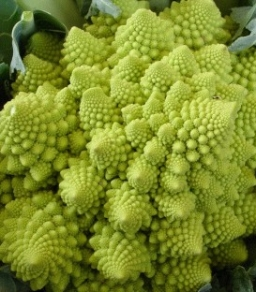Did you find an error or inaccuracy? Feel free to write us. Thank you!Tips to related online calculators
Need help to calculate sum, simplify or multiply fractions? Try our fraction calculator.

## Related math problems and questions:

• 6/24 change6/24 change to a fraction in lowest terms
• Reciprocal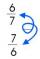Calculate the reciprocal numbers for the given real numbers.
• Reciprocals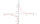Which among the given reciprocal is correct a. 3/15x1/3= 1 b. 3/20x20/3=1 c. 7/14x7/7=1 d. 34/3x34/34=1
• Change the numbers in the ratio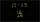Change the numbers 29, 38, and 43 in a 3: 4 ratio.
• Reciprocal equation 3Solve reciprocal equation: 1/2 + 2/3=1/x
• Oil change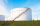A container holds 1/4 gallon of oil. Jack is changing the oil in his vehicles and needs 11 containers. How many gallons of oil does Jack need?
• Equivalent fractions 2Write the equivalent multiplication expression. 2 1/6÷3/4
• ReciprocalCalculate reciprocal of z=0.8-1.8i:
• Numbers divisionWith what number should be divided mixed number 2 3/4 to get 11/12?
• Dividing walnuts into crates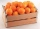There are 8 and 2 over 3 pounds of walnuts in a container, which will be divided equally into containers that hold 1 and 1 over 5 pounds. This would fill n and 4 over 18 containers. What is n?
• Reciprocal value 2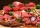Annabelle is making burger patties if each burger patty needs 1/16 kilogram of beef. How many patties can she make with a kilo of beef?
• Simplest form 3What fraction is 15 of 35 in simplest form?
• Dividing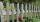Divide the three-line segment 13 cm, 26 cm, and 19.5 cm long for parts so that the individual pieces were equally long and longest. How long will the individual parts, and how many will it?
• Self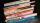A math text book is 2 2/9 inches thick. how many of these books will fit on a 120-inch self?
• Divide 11Divide the product of 4 and 5/8 by 1 1/2. Write your answer as a mixed number.
• Two numbers and its productThe product of two numbers are 2/3. If on of them is 1/10, what is the other?
• Two divided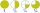Two divided by nine-tenths.